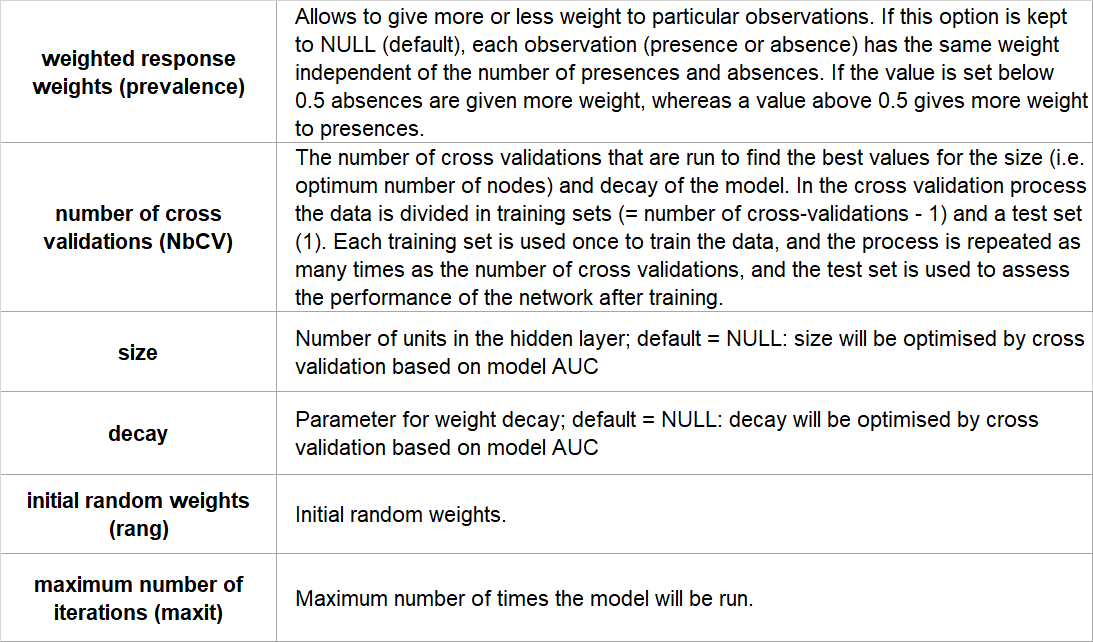### Introduction

The term Artificial Neural Networks (ANN) refers to a large group of models that are inspired by biological neural networks, in particular the brain, which consists of extremely large interconnected networks of neurons to process information. Similarly, Artificial Neural Networks consist of a large number of nodes and connections. These are typically organised in layers, with an input layer in which the data is fed into the model, a number of hidden layers, and the output layer which represents the result of the model. We focus here on single hidden layer feed-forward Artificial Neural Networks that are trained by back-propagation, which are the most commonly used ANNs in ecology.The input layer consists of the environmental data that are put in the model, with each input node representing one environmental variable. The information from each node in the input layer is fed into the hidden layer. The connections between the nodes in the input layer and the nodes in the hidden layer can all be given a specific weight based on their importance. These weights are usually randomly assigned at the start of the model, but the model can learn and optimize the weights in subsequent runs in the back-propagation process. The higher the weight of a connection, the more influence that particular input node has. The nodes in the hidden layer are thus comprised of different combinations of the environmental variables, and they receive the information from the input layer in a way in which the input is multiplied by the weight of the connection and summed. This calculation is done for each node in the hidden layer. The weighted sums in each of the hidden layer nodes are passed into a so-called ‘activation function’, which transforms the weighted input signal into an understandable output signal.There are a lot of different forms of activation functions, but a commonly used one is the logistic function that produces a sigmoid curve with an outcome between 0 and 1. The outcome of the activation function is then passed on to the output layer. Similar to the connections between the input and hidden layers, the connections between the hidden layers and the output layer are weighted, and thus the output is the result of the weighted sum of the hidden nodes. In a species distribution model the output layer is the prediction whether a species will be present or absent in a given location.As part of the training of the model, the output is compared to the desired output. In a species distribution model, the desired output is based on the known occurrence locations and the environmental conditions of those locations. The difference between the predicted outcome of the model and the desired outcome is the error of the model, and this is used to improve the model in the back-propagation process. In this process the weight of each connection is recalculated by multiplying the old weights by the difference between the output from the model and the desired output. Based on these new weighted connections, the nodes in the hidden layer can calculate their own error, and use this to adjust the weights of the connections to the input layer. After all the weights have been adjusted, the model recalculates the output in the feed forward way, so starting again from the input layer through the hidden layer to the output. This process is repeated several times until the model reaches a pre-defined accuracy, or a maximum set number of runs.

• High predictive power
• Able to handle large datasets
• Able to model non-linear associations between the response and the predictors

### Limitations

• Sensitive to missing data and outliers
• Less efficient in handling data of ‘mixed types’
• Time-consuming

### Assumptions

No formal distributional assumptions (non-parametric).

Yes.

### Configuration options

BCCVL uses the ‘nnet’ package, implemented in biomod2. The user can set the following configuration options:### References

• Franklin J (2010) Mapping species distributions: spatial inference and prediction. Cambridge University Press.
• Lek S, Guégan JF (1999) Artificial neural networks as a tool in ecological modelling, an introduction. Ecological modelling, 120(2): 65-73.
• Olden JD, Jackson DA (2002) Illuminating the “black box”: a randomization approach for understanding variable contributions in artificial neural networks. Ecological modelling, 154(1): 135-150.
• Olden JD, Lawler JJ, Poff NL (2008) Machine learning methods without tears: a primer for ecologists. The Quarterly review of biology, 83(2): 171-193.